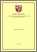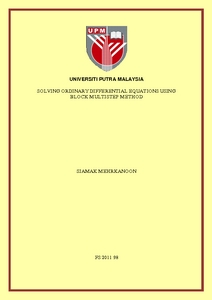# Solving ordinary differential equations using block multistep method

## Citation

Mehrkanoon, Siamak (2011) Solving ordinary differential equations using block multistep method. PhD thesis, Universiti Putra Malaysia.

## Abstract

An eficient code based on block multistep method is developed for solving first and higher order initial value problems (IVPs) of ordinary differential equations (ODEs) using variable step size strategy. Unlike previous methods, the Gauss-Seidel approach is used for the implementation of the proposed method instead of Jacobi iteration. Therefore by applying the current technique, the desired order of accuracy can be achieved with less number of function evaluation, since the latest available information is used to advance the numerical solution through a given step size. The higher order IVPs are solved directly without reducing it into the first order IVPs. In addition, the stability of the proposed methods are discussed. Furthermore, the parallel version of the proposed methods are constructed in order to solve large system of first and second order of IVPs. The parallelism across the system is considered for the implementation of the parallel block methods. Less computational time is required when the parallel versions are applied compared to sequential methods. The parallel implementation is supported by Message Passing Interface (MPI). Both sequential and parallel algorithms were carried out on Sunfire V1280 with eight homogeneous processors located at Institute of Mathematical Research (INSPEM), University Putra Malaysia. Subsequently, a variable block method is proposed to solve first and higher order IVPs of ODEs using variable step size and order strategy. In previous researches, in order to increase the order of the method, it supposed to use the information of more back points. In the proposed technique, attempts have been made to promote the order of the method via involving the advanced points. By utilizing the present code we are able to not only increase the order of the method but also to reach the end of the given interval faster. In conclusion, the developed new codes deserve to be used for solving system of first and higher order IVPs of ODEs.Preview
PDF
FS 2011 98R.pdfView Item